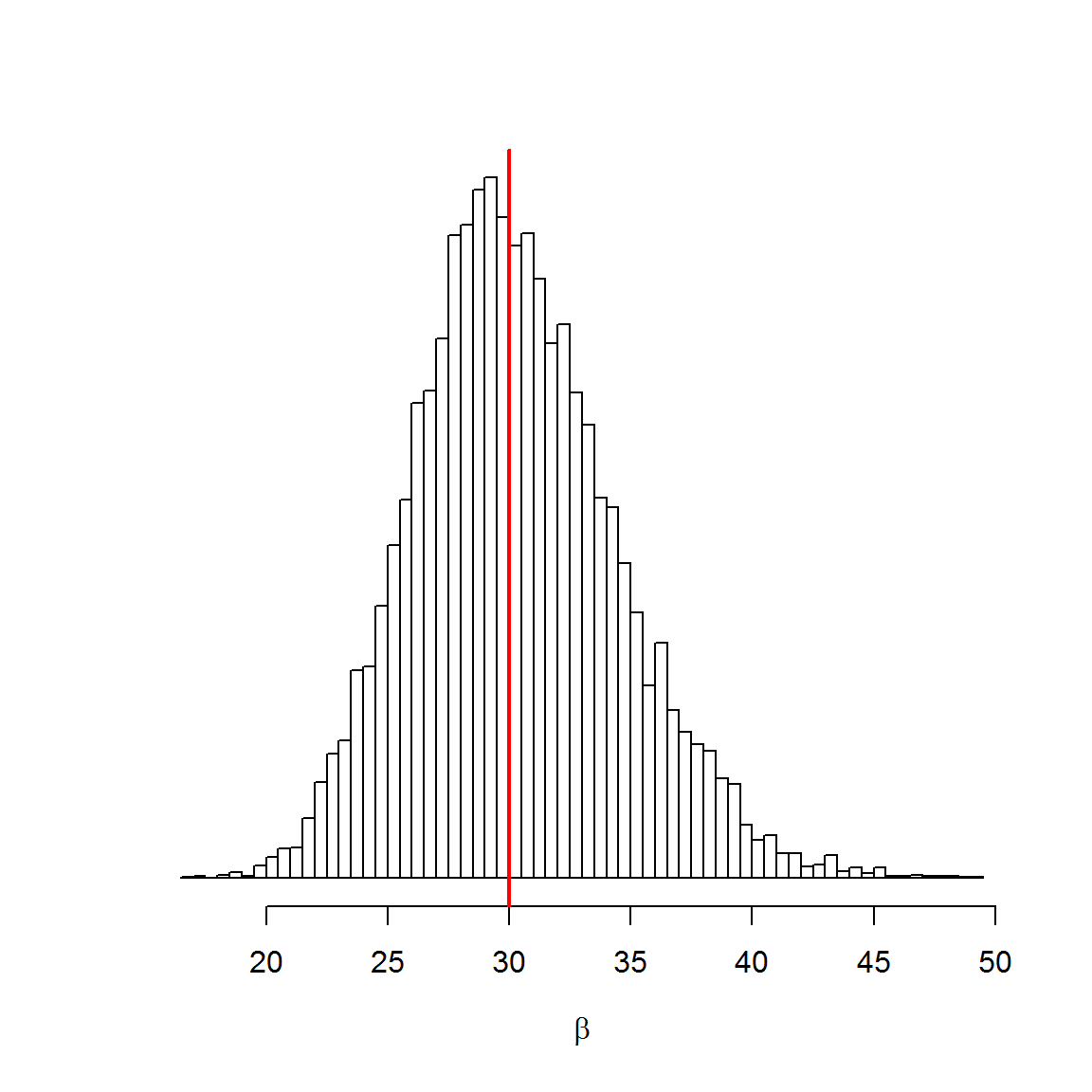# Introduction

Refs:

This problem was first presented by Stephen F. Gull in 1988 and can be stated as:

A lighthouse is somewhere off a piece of straight coastline at a position $$\alpha$$ along the shore and a distance $$\beta$$ out at sea. It emits a series of short highly collimated flashes at random intervals and hence at random azimuths. These pulses are intercepted on the coast by photo-detectors that record only the fact that a flash has occurred, but not the angle from which it came. N flashes have so far been recorded at positions {$$x_k$$}. Where is the lighthouse?’ – Sivia, pg.29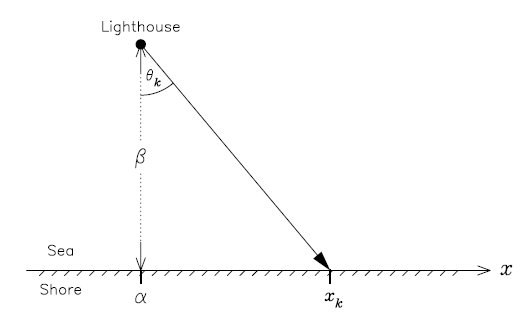Problem diagram (also from Sivia)

As implied in the description, the angle $$\theta$$ is uniform over $$\pm \pi/2$$, so

$p(\theta | \alpha, \beta) = \frac{1}{\pi}$

The position $$x$$ is given by

$x = \beta \tan \theta + \alpha$

The rule of variable transformation, $$p(x) dx = p(\theta) d\theta$$, allows us to compute $$p(x | \alpha, \beta)$$:

$p(x | \alpha, \beta) = p(\theta | \alpha, \beta) \left| \frac{d\theta}{dx} \right|$

Since $$x = \beta \tan \theta + \alpha$$, if we differenciate $$\theta$$ wrt $$x$$:

$\frac{d\theta}{dx} = \beta \sec^2\theta = \beta (\tan^2\theta + 1) = \beta \left[\left(\frac{x-\alpha}{\beta}\right)^2 + 1\right] = \frac{\beta}{(x-\alpha)^2 + \beta^2}$

So, getting back to $$p(x | \alpha, \beta)$$:

$p(x | \alpha, \beta) = p(\theta | \alpha, \beta) \left| \frac{d\theta}{dx} \right| = \frac{1}{\pi} \frac{\beta}{(x-\alpha)^2 + \beta^2}$

This distribution is precisely the Cauchy distribution. It has two parameters, $$x_0$$, location (which we called $$\alpha$$, above), and $$\beta>0$$, scale:

$p(x|x_0,\beta) = \frac{1}{\pi} \frac{\beta}{(x-x_0)^2 + \beta^2}$

Notice that this is a two paramater problem, $$\alpha$$ and $$\beta$$.

# Known $$\beta$$

First, let’s consider we know $$\beta$$, the distance from shore, and we wish to find

$p(\alpha | \{x_k\}, \beta)$

Using Bayes’ theorem:

$p(\alpha | \{x_k\}, \beta) \propto p(\{x_k\} | \alpha, \beta) p(\alpha | \beta)$

and since $$\alpha \perp \beta$$, $$p(\alpha | \beta) = p(\alpha)$$.

The prior $$p(\alpha)$$ can be modelled by a uniform over a large enough segment $$[x_{min}, x_{max}]$$, ie, $$p(\alpha) = 1/(x_{max}-x_{min})$$.

For the data likelihood $$p(\{x_k\} | \alpha, \beta)$$ we consider that each datum $$x_k$$ is iid, so:

$p(\{x_k\} | \alpha, \beta) = \prod_{k=1}^N p(x_k | \alpha, \beta)$

And since the prior for $$\alpha$$ is uniform, we finally get:

$p(\alpha | \{x_k\}, \beta) \propto p(\{x_k\} | \alpha, \beta)$

Let’s try some R.

The next function is a factory that creates the log posterior function for a given data:

# outputs function log p(alpha|x_k,beta)
#
# computes the cauchy pdf for the likelihood p(x_k|alpha,beta), since the prior(alpha)
# is constant and does not contribute to find the posterior distribution shape
make_log_posterior <- function (x_k, beta) {
Vectorize(function (alpha_hat) {
sum( log((beta/pi) / (beta^2 + (x_k - alpha_hat)^2)) )
})
}

Let’s create a dataset:

alpha <- 10 # unkonwn true values
beta  <- 30 # this is known for now
##################
set.seed(123)
N       <- 1024
theta_k <- runif(N,-pi/2,pi/2)
x_k     <- beta * tan(theta_k) + alpha

And check it:

log_posterior <- make_log_posterior(x_k, beta)
curve(log_posterior, from=-25, to=25, n=500, xlab=bquote(alpha), ylab="")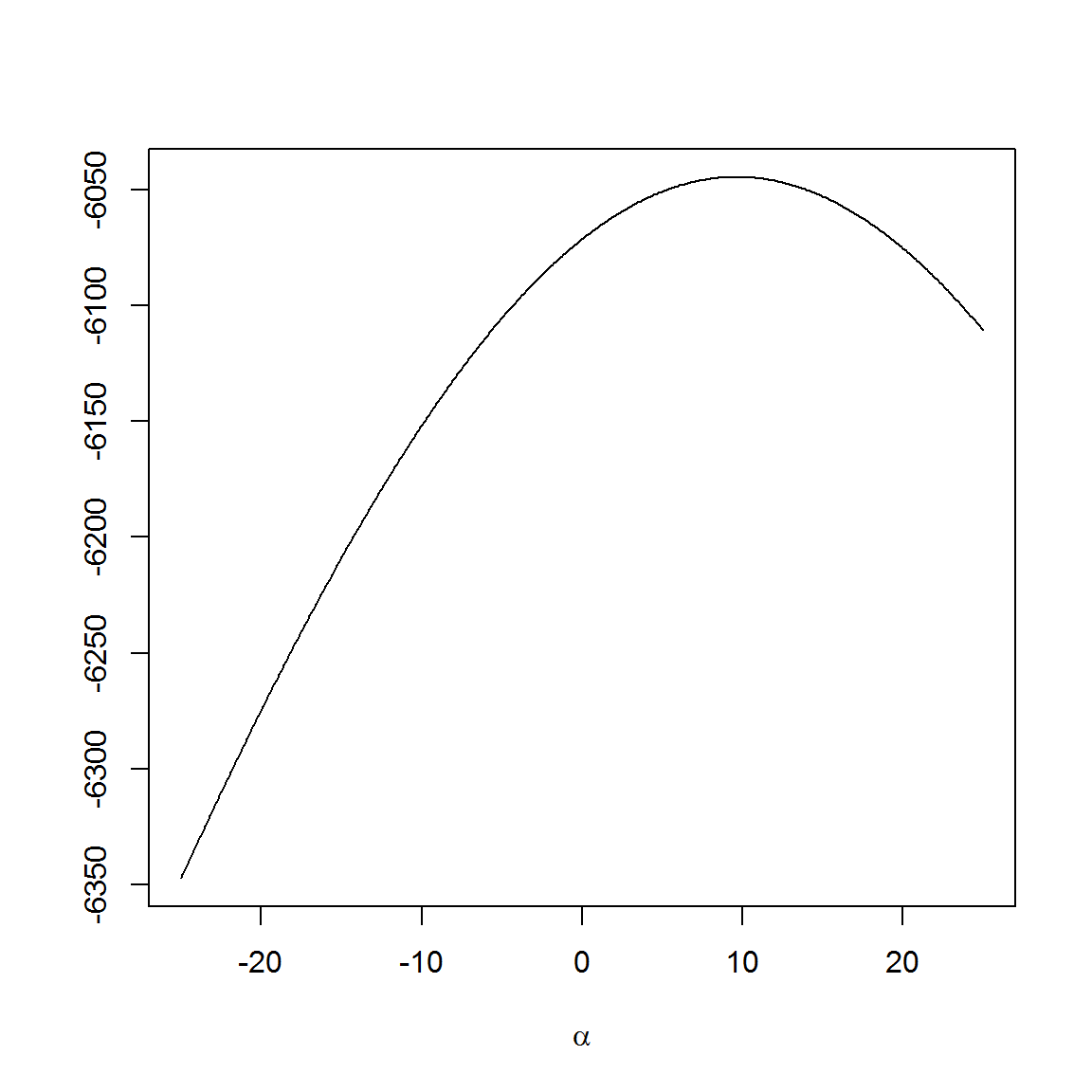We see that there’s a maximum over there, but we are working with logs (btw, we must work with logs to make sums, since working directly with products would easily result in under/overflows).

After the logs are computed, we can get the shape of the posterior (to get the true posterior – a distribution – we would need to find the normalizing factor, which is irrelevant here):

posterior_shape <- function(xs, log_posterior) {
log_alphas    <- log_posterior(xs)        # compute log posterior for a grid of values
log_alpha_max <- max(log_alphas)
alphas <- exp(log_alphas - log_alpha_max) # subtract from L_max, and exponentiate
alphas                                    #  then the max value will be 1
}

xs <- seq(0,20,len=1000)
plot(xs, posterior_shape(xs, log_posterior), type="l", xlab=bquote(alpha), ylab="")
abline(v=alpha, col="grey40", lty=2)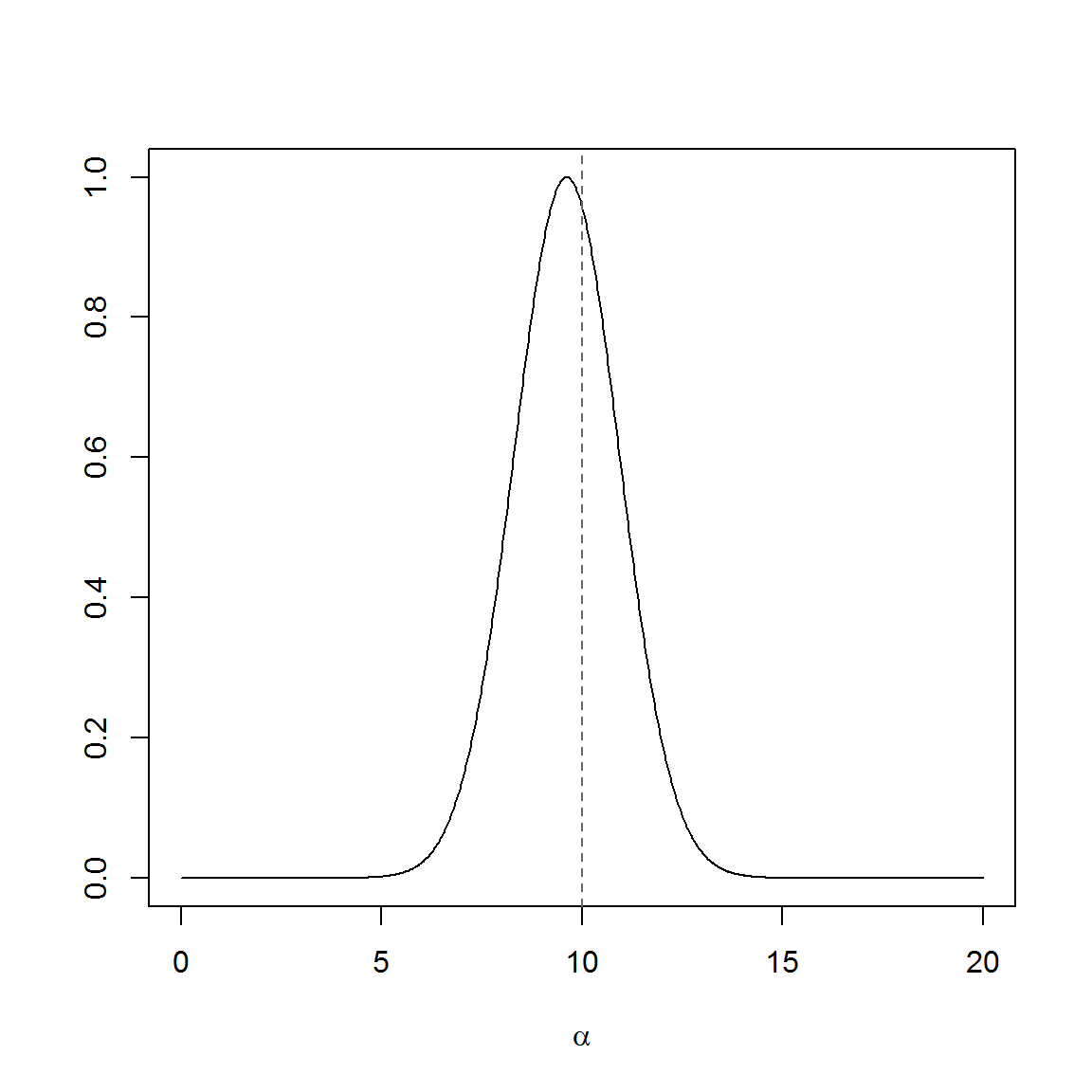The likelihood is given by a Cauchy, which is a symmetric distribution, and it would be natural to assume that the mean would be a good estimator. However:

mean(x_k)
##  -17.72149

The mean is not a good estimator because we cannot apply the Central Limit Theorem (CLT). The CLT only works when the data is iid (which it is) and sampled from a distribution with finite mean (which it is not). The Cauchy does not have a mean.

The Cauchy does have a median, $$x_0$$, which can be used as an estimator here:

median(x_k)
##  8.964787

but does not give as much information as the posterior does.

The posterior might be multimodal when the data is sufficiently appart:

x2_k <- c(0,0,200,400,400)
log_posterior2 <- make_log_posterior(x2_k, beta)

xs <- seq(-100,500,len=1000)
plot(xs, posterior_shape(xs, log_posterior2), type="l", xlab=bquote(alpha), ylab="")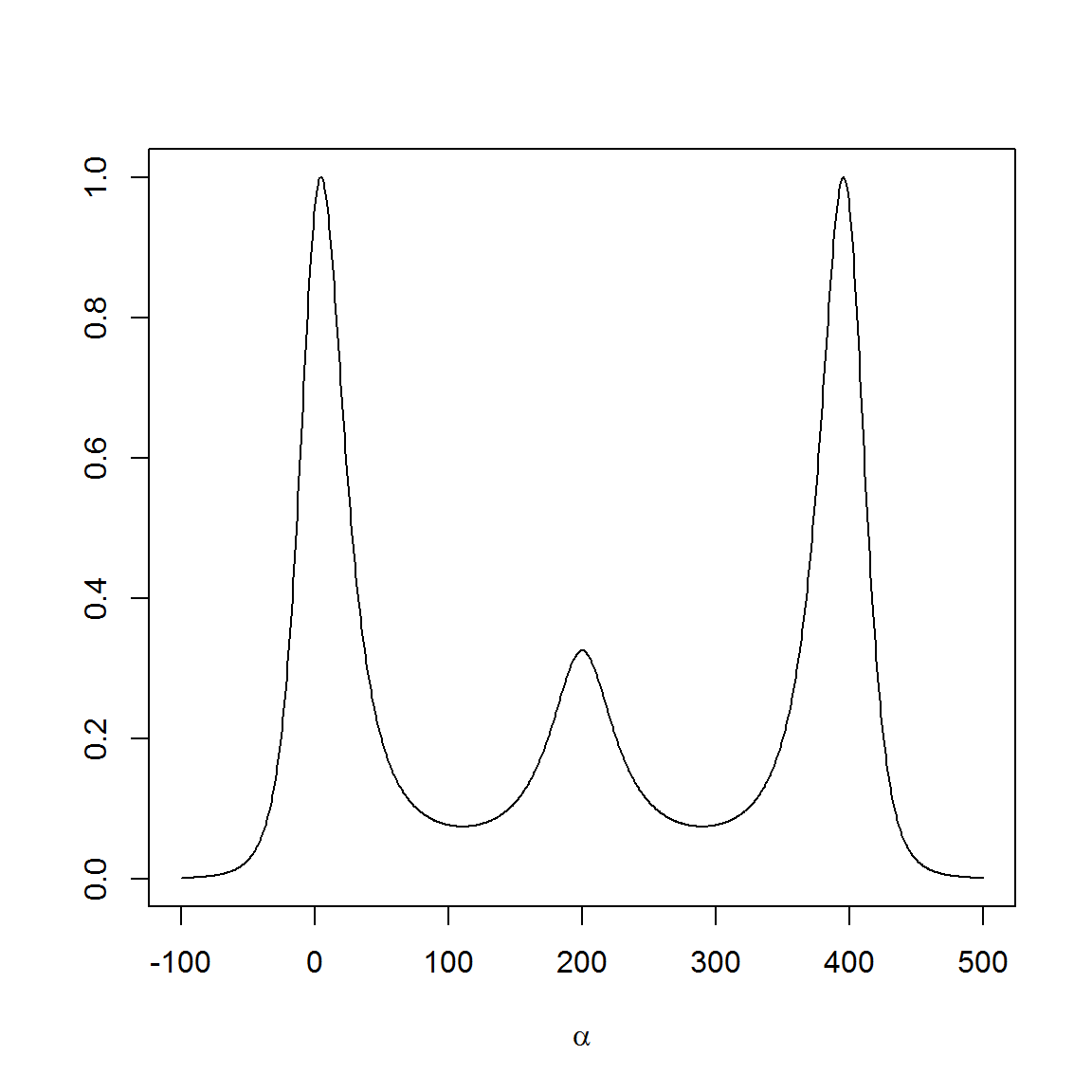# Unknown $$\beta$$

If we also don’t know $$\beta$$ then we have to add a prior to it.

Let’s assume $$\alpha \perp \beta$$, so we get:

$p(\alpha, \beta | \{x_k\}) \propto p(\{x_k\} | \alpha, \beta) p(\alpha) p(\beta)$

If we also assume that $$\beta$$ is uniform over some interval [$$0,y_{max}$$], then the posterior is proportional to the likelihood:

$p(\alpha, \beta | \{x_k\}) \propto p(\{x_k\} | \alpha, \beta)$

We can use R to check how the posterior would look like for our previous eg:

log_joint_posterior <- function (x_k, alfa, beta) {
sum( log((beta/pi) / (beta^2 + (x_k - alfa)^2)) )
}

And check our sample dataset using a grid search:

x_min <- 0; x_max <- 20; y_max <- 50
alphas <- seq(x_min, x_max)
betas  <- seq(    0, y_max)

f <- function(a,b) log_joint_posterior(x_k, a, b)

log_grid_values <- outer(alphas, betas, Vectorize(f))

grid_values <- matrix(posterior_shape(log_grid_values, log_posterior),
nrow=length(alphas), ncol=length(betas))

This requires a 3D plot:

persp(alphas, betas , grid_values,
xlab=bquote(alpha), ylab=bquote(beta), zlab="",
main="" , cex=0.7, lwd=0.1  ,
xlim=c(x_min,x_max), ylim=c(0,y_max), zlim=c(0,1),
title(bquote(paste(p,"(",alpha,",",beta,"|",x[k],")")))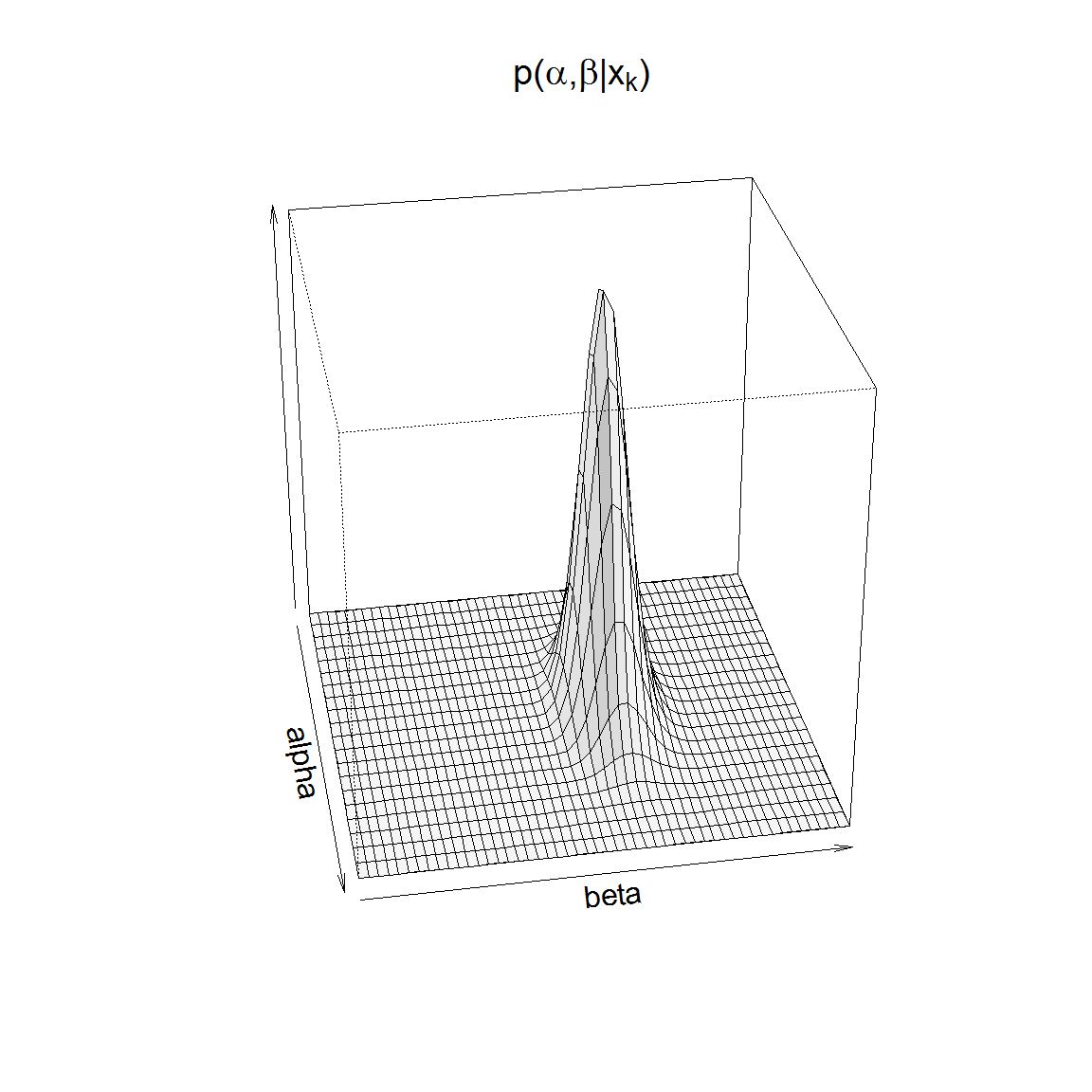Or a contour map:

contour(alphas, betas, grid_values, xlim=c(4,15), ylim=c(20,40))
points(alpha, beta, pch=3, col="blue", lwd=2) # true value
legend("topright",c("true position"), col="blue", pch=3, pt.cex=1.2, pt.lwd=2)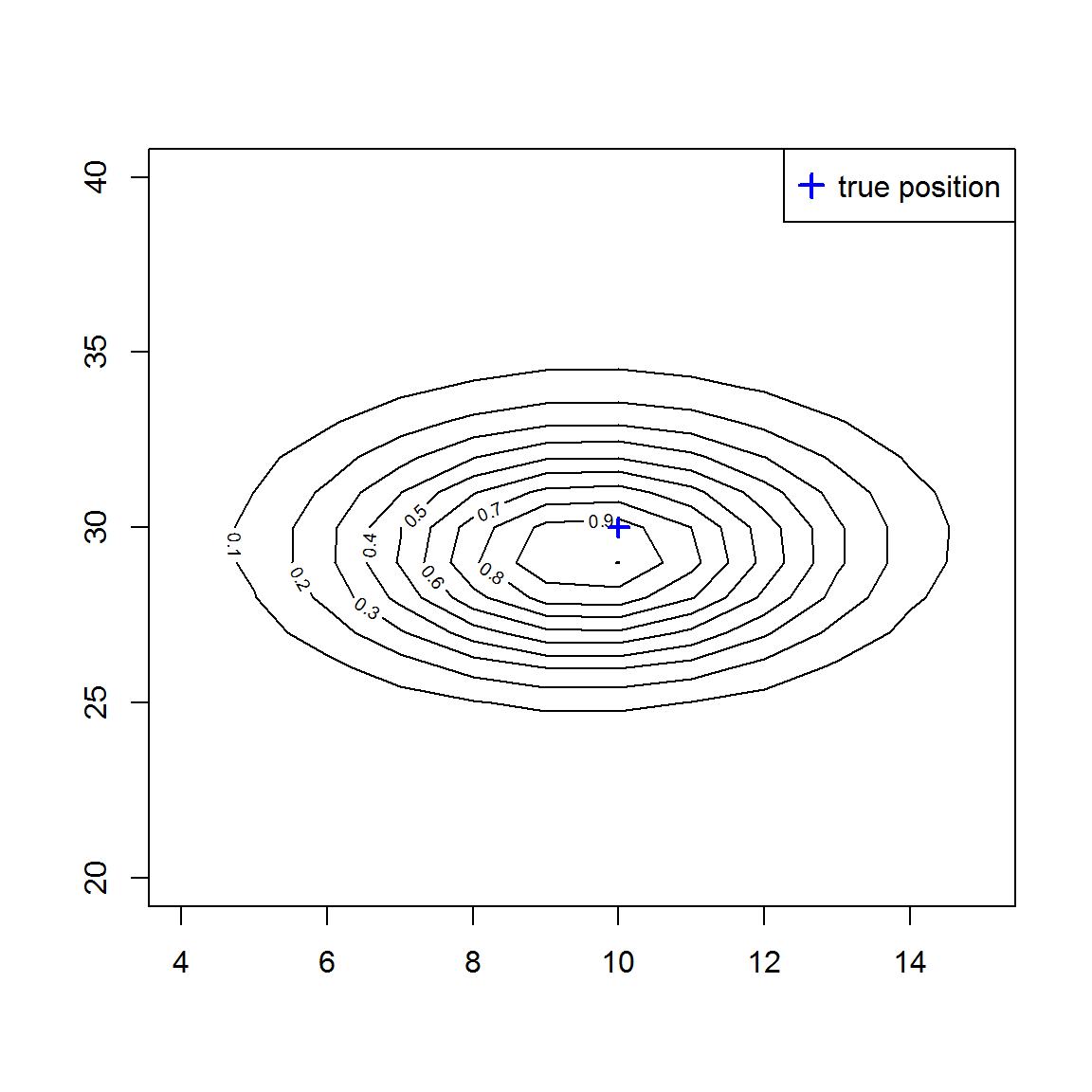## Using Stan

library(rstan)

The next Stan program models our problem (adapted from here):

model <- '
data {
int<lower=0> N;
real x_[N];
}

parameters {
real alpha;
real<lower=0> beta;
}

model {
alpha ~ uniform(0, 20);
beta  ~ uniform(0, 50);

for (k in 1:N) {
x_[k] ~ cauchy(alpha, beta);
}
}
'

Let’s compile and run the model in Stan:

n <- 100  # get a subset of the data
stan_input <- list(x_=x_k[1:n], N=n)

fit  <- stan(model_code=model, data=stan_input, iter=1000, verbose=FALSE)
fit2 <- stan(fit=fit, data=stan_input, iter=5000, warmup=2000, verbose=FALSE)
print(fit2, pars=c("alpha", "beta"))
## Inference for Stan model: 36eef3c3637fb3a9564529926f8463fe.
## 4 chains, each with iter=5000; warmup=2000; thin=1;
## post-warmup draws per chain=3000, total post-warmup draws=12000.
##
##        mean se_mean   sd  2.5%   25%   50%   75% 97.5% n_eff Rhat
## alpha  8.96    0.05 3.91  1.61  6.13  8.95 11.68 16.80  7079    1
## beta  30.29    0.05 4.18 22.84 27.45 29.98 32.88 39.18  8470    1
##
## Samples were drawn using NUTS(diag_e) at Wed Jan 11 17:25:35 2017.
## For each parameter, n_eff is a crude measure of effective sample size,
## and Rhat is the potential scale reduction factor on split chains (at
## convergence, Rhat=1).

These are the empirical posteriors (the vertical red line is the true parameter value):

la <- extract(fit2)
alpha_hats <- as.numeric(la$alpha) beta_hats <- as.numeric(la$beta)
hist(x=alpha_hats, breaks=100, xlab=bquote(alpha), ylab="",main="", yaxt='n')
abline(v=alpha, col="red", lwd=2)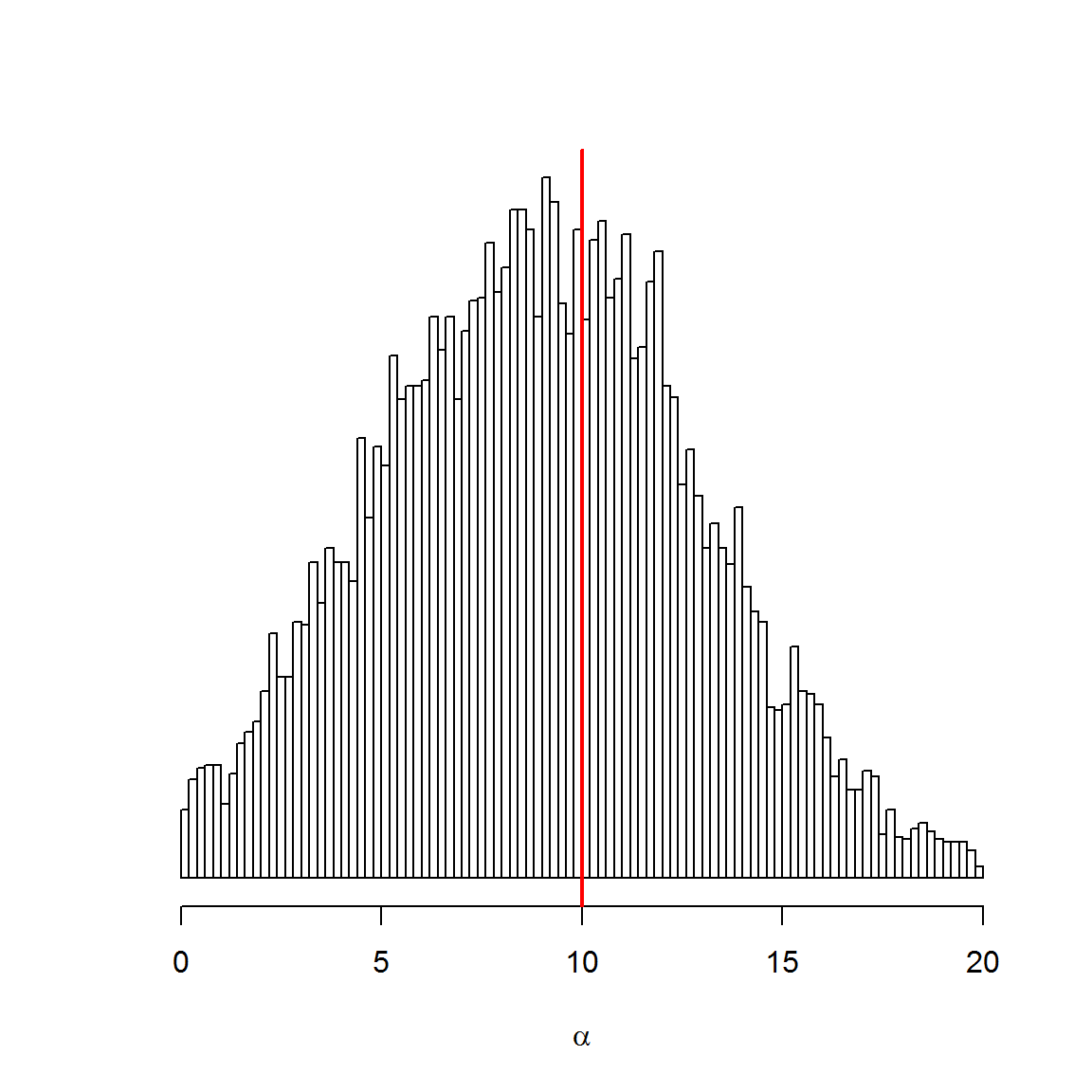hist(x=beta_hats, breaks=100, xlab=bquote(beta), ylab="",main="", yaxt='n')
abline(v=beta, col="red", lwd=2)